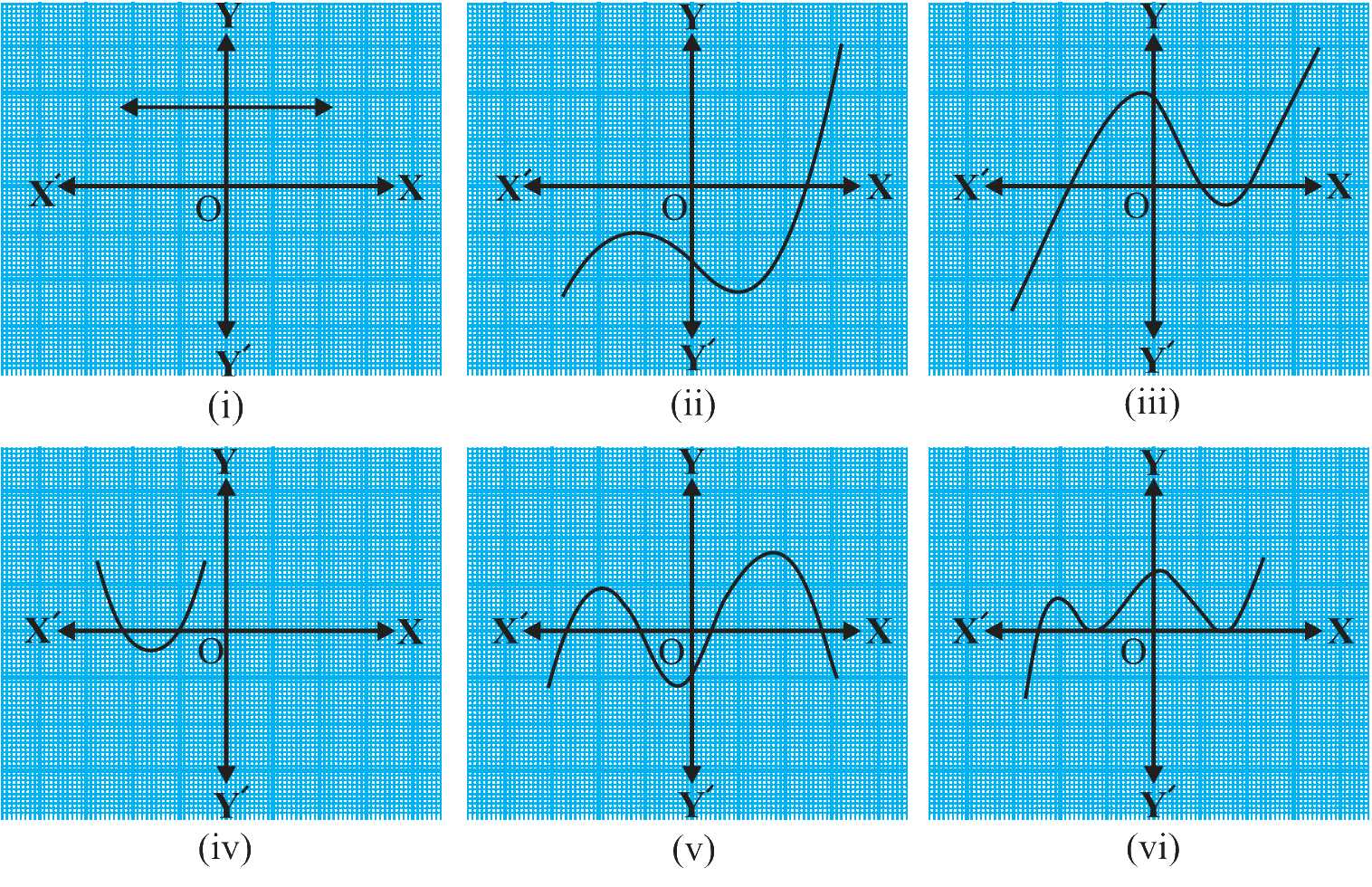NCERT Class 10 Maths Polynomials

# NCERT Class 10 Maths Polynomials

The chapter 2 begins with the revision of the concept of the degree of a polynomial - the highest power of x in p(x). Also, linear, quadratic and cubic polynomials are introduced. Next, the concept of the zero of a polynomial is explained. Further, the geometric meaning of the zeroes of the polynomial is covered. Next, the relationship between Zeroes and Coefficients of a Polynomial is taken up for explanation, which is then followed by several solved examples. Thereafter, for the third exercise, the Division Algorithm for polynomials is presented and multiple examples are provided so that students can gain a deeper understanding of the procedure.

Download FREE PDF of Chapter-2 Polynomials

## Chapter 2 Ex.2.1 Question 1

The graphs of $$y{ }={ }p\left( x \right)$$ are given in the figure below, for some polynomials $$p\left( x \right)$$. Find the number of zeroes of $$p\left( x \right)$$ in each case.### Solution

What is known?

The graphs of $$y{ }={ }p\left( x \right)$$ are given in the above figure for some polynomials $$p\left( x \right)$$.

What is unknown?

The number of zeroes of $$p\left( x \right)$$ in each case.

Reasoning:

You can reach the solution easily by understanding the statement of the question. As The graphs of $$y{ }={ }p\left( x \right)$$ are given and you have to find the number of zeroes of $$p\left( x \right)$$ in each case.

To find this look at the graphs and visually find how many points it cuts the $$x$$-axis. The number of points the graph cuts or touches the $$x$$-axis. The number of points it cut or touches the $$x$$-axis are the zeroes of the polynomial $$p\left( x \right)$$.

Steps:

(i) The number of zeroes is $$0$$ as the graph doesn’t cuts the $$x$$-axis at any point.

(ii) The number of zeroes is $$1$$ as the graph cuts the $$x$$-axis at only one point.

(iii) The number of zeroes is $$3$$ as the graph cuts the $$x$$-axis at $$3$$ points.

(iv) The number of zeroes is $$2$$ as the graph cuts the $$x$$-axis at $$2$$ points.

(v) The number of zeroes is $$4$$ as the graph cuts the $$x$$-axis at $$4$$ points.

(vi) The number of zeroes is $$3$$ as the graph cuts the $$x$$-axis at  $$3$$ points.

Polynomials | NCERT Solutions
Instant doubt clearing with Cuemath Advanced Math Program